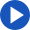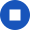## calculate

Regular
Change speakerYou're listening to Joanna
She has an American accent and expects you to use American spelling.

•••••Past

• I calculated
• you calculated
• he/she/it calculated
• we calculated
• you calculated
• they calculated

Present

• I calculate
• you calculate
• he/she/it calculates
• we calculate
• you calculate
• they calculate

Future

• I will calculate
• you will calculate
• he/she/it will calculate
• we will calculate
• you will calculate
• they will calculate

JoannaYouReset